Meta-Heuristic Algorithms for Solving Hydrothermal System Scheduling Problem Considering Constraints in Transmission Lines | OMICS International
Global Journal of Technology and Optimization

# Meta-Heuristic Algorithms for Solving Hydrothermal System Scheduling Problem Considering Constraints in Transmission Lines

 Thang Trung Nguyen1,2, Dieu Ngoc Vo3*, Anh Viet Truong2 and Loc Dac Ho3 1Faculty of Electrical and Electronics Engineering, Ton Duc Thang University, HCM City, Vietnam 2Faculty of Electrical and Electronics Engineering, University of Technical Education HCM City, HCM City, Vietnam 3Department of Power Systems, Ho Chi Minh City University of Technology (HUTECH), HCM City, Vietnam *Corresponding Author : Dieu Ngoc Vo Department of Power Systems Ho Chi Minh City University of Technology (HUTECH) HCM City, Vietnam Tel: 84-838647256 E-mail: [email protected] Received: April 06, 2016 Accepted: April 27, 2016 Published: April 29, 2019 Citation: Nguyen TT, Vo DN, Truong AV, Ho LD (2016) Meta-Heuristic Algorithms for Solving Hydrothermal System Scheduling Problem Considering Constraints in Transmission Lines. Global J Technol Optim 7:192. doi:10.4172/2229-8711.1000192 Copyright: © 2016 Nguyen TT, et al. This is an open-access article distributed under the terms of the Creative Commons Attribution License, which permits unrestricted use, distribution, and reproduction in any medium, provided the original author and source are credited.

Visit for more related articles at Global Journal of Technology and Optimization

#### Abstract

This paper presents the applications of Cuckoo search algorithm (CSA) for solving the problem of optimal power flow for hydrothermal system (OPF-HTS) where IEEE 30-bus test system with both thermal plants and hydropower plants is considered. The problem is first developed in the paper by the combination of optimal power flow (OPF) problem and short-term hydrothermal scheduling (STHTS) problem and it becomes much more complicated than the two sub-problems because it includes all constraints of transmission grid from the comer and all hydraulic constraints from the later in addition to the multi optimal subintervals. In order to validate the performance of the CSA when applied to the problem, another existing meta-heuristic algorithm, Particle swarm optimization has been employed to solve the same problem and compare the obtained results. The analysis on the obtained results has indicated that the CSA is more effective and robust than PSO. Consequently, it can be sated that CSA is a very efficient method for solving the problem.

 Keywords Cuckoo search algorithm; Particle swarm optimization; Optimal power flow; Hydrothermal system Introduction Optimal power flow (OPF) is a static non-linear programming problem where the main task is to determine steady state operation points of all generators available in the power systems so that the total fuel cost is minimized as much as possible while exactly meeting a set of physical and operational constraints imposed by equipment and network limitations such as active and reactive power of generators, transformer tap, switchable capacitor bank, bus voltage, and transmission line capacity limits . Generally, the controllable variables of the OPF problem first need to be determined are active powers of generators, voltage magnitude at generation buses, injected reactive power at capacitor buses, and transformer tap settings and then other dependent variables are obtained such as reactive power of the generators, voltage magnitude at other buses, etc. Objective of a conventional OPF problem is only to minimize total fuel cost whereas other objective such as power losses in transmission lines, emission released into the air by thermal power plants using fossil fuels as well as voltage stability index are neglected. Consequently, the objective of the OPF problem is extended to minimize the several objectives consisting of fuel cost, transmission losses, emission and voltage stability index. Hydrothermal system is comprised of both thermal plants and hydropower plants supplying electricity to load via transmission lines. The system has become popular in power system due to their significant contribution to power source since renewable energy cannot afford enough power energy to electrical load. It is derived from connection of the both types of plants in order to keep power system working stably and economically, the cooperation of the hydrothermal system has played an important role. The operation of the hydrothermal system is far more complicated as hydropower plants have neglected fuel cost due to its free water from nature rivers, but is required to operate under the constraint of hydraulic reservoirs and water available for hydro generation in a given scheduled time period. On the contrary to hydropower plants, the fuel cost for power generation is the main objective during operation of thermal plants but the set of constraints taken into account is much simple once only limitations on thermal generation is included. Therefore, hydrothermal system scheduling (HTSS) aims to minimize electricity generation fuel cost of thermal plants using fossil fuels while all constraints from thermal plants and hydropower plants as well as power balance constraint from power grid must be exactly met. The thermal plant constraint is simple to deal with since only limitations on generations are taken into consideration and other constraints such as fossil fuel constraint, fuel cost for start-up and shutdown process is neglected. On the contrary, hydropower plant constraints are much more complicated because the operation of the plant includes not only generators but also reservoirs, which must satisfy hydraulic constraints and the aim of agriculture water. The two problems, optimal power flow and hydrothermal system scheduling have been separated into two different problems so far because of their complex. In fact, HTSS problem takes hydraulic constraints over a number of optimal subintervals into consideration meanwhile the OPF problem considers a large set of variables in power grid consisting of transmission capacity, voltage at all buses and reactive and active power of generators, etc. In fact, many so-far articles divide the large scale problem of multi-objective optimal power flow for hydrothermal systems into two problems including multi-objective optimal power flow problem [2-9] and multi-objective hydrothermal scheduling problem [10-16]. Unfortunately, there have not been articles so far regarding to both OPF and HTS problems. Consequently, a new problem is first developed in the study by combining hydrothermal system and the power grid, called optimal hydrothermal system power flow (OHTSPF). In the problem, the main task is to minimize several objectives including fuel cost of the first HTS problem and power losses of the second OPF problem while satisfying all constraints from the first HTS problem consisting of water discharge, available amount of water and limitations on generators, and constraints on the power grid from the second OPF problem such as reactive power of generators, transformer tap, switchable capacitor bank, bus voltage, and transmission line capacity limitations. Cuckoo search algorithm (CSA) is a recently developed metaheuristic algorithm inspired from the cuckoo bird’s reproduction behavior . In World Congress on Nature and Biologically Inspired Computing (NaBIC) , ten benchmark optimization functions with the dimension up to 256 have been used to test the ability of CSA, genetic algorithm (GA) and particle swarm optimization (PSO). The obtained results have shown that the CSA can obtain not only lower average number of function evaluations and standard deviation than both GA and PSO but also 100% successful rate, which is much higher than that of GA and PSO. In this paper, the CSA is applied for solving the problem of MOOPF- HTS. In addition, another meta-heuristic algorithm, Particle swarm optimization is also employed to find out the optimal solution, which is verified if all constraints from hydrothermal system and power gird are satisfied. Furthermore, the obtained results in terms of fuel cost of thermal plants and emission from the applied CSA and PSO are compared to specify a better algorithm and it will be suggested for application to the problem. Problem Formulation Objective function Fuel cost objective: The detailed OPF problem is formulated as follows: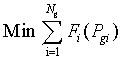(1) where the fuel cost function Fi(Pgi) of generating unit i can be expressed in one of the forms as follows: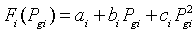(2) where ai, bi and ci are the fuel cost coefficients and Pgi is the power output of thermal plant i. Emission objective: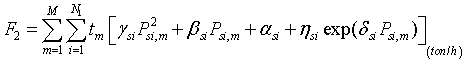(3) where αsi, βsi, γsi, ηsi, and δsi are emission coefficients of thermal unit i. Power loss objective: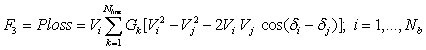(4) where Vi, δi and Vj, δj are voltage magnitude and angle at bus i and j, respectively; Gk is a conductance of the kth line; Nline is the number of transmission lines. Nb is the number of buses. Hydrothermal system constraints Load demand: The total power generation from thermal units and hydro units at generation buses must satisfy the load demand at load buses and total power losses in transmission lines represented by: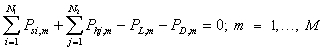(5) Water availability constraints: The total water discharge for each hydro unit during the scheduled period is limited by the available amount of water for that unit as follows: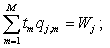j = 1, …, N2 (6) where qj,m is the rate of water flow via turbine of hydro plant j in interval m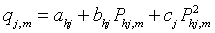(7) where ahj, bhj, chj are water discharge coefficients of hydro unit j and Wj is the volume of water available for generation by hydro plant j during the scheduled period. Generator operating limits: The power output of thermal and hydro units is limited between their upper and lower limits; however, in the problem, each unit has limitations of active and reactive power and included at generation buses.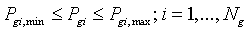(8)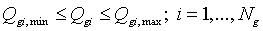(9) In addition, each generation bus must be satisfied the constraint of voltage as below.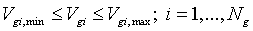(10) Transmission grid constraints • Real and reactive power flow equations: At each bus, the real and reactive power balance should be satisfied.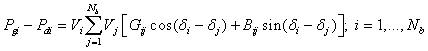(11)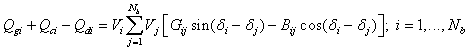(12) where Pdi, Qdi are real and reactive power demands at bus i, respectively; and Pgi, Qgi are real and reactive power outputs of generating unit i, respectively. • Capacity limits for switchable shunt capacitor banks: At buses where capacitors are located to supply reactive power to the grid must be exactly met the limitations as follows.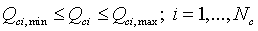(13) • Transformer tap settings constraints: The tap of transformers during working condition must be within its range as the following inequality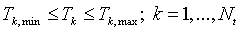(14) • Voltage constraint for load buses: The voltage at load buses must be in limitation of working values determined by: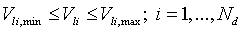(15) • Power flow constraints: the apparent power between two buses must be lower or equal to the maximum capacity of conductor.• Power flow constraints: the apparent power between two buses must be lower or equal to the maximum capacity of conductor.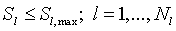(16) where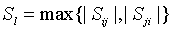(17) Sets of Variables A Set of control variables From the problem formulation, u is the set of control variables (independent variables) including real power output of generators at generation buses except the slack bus Pgi with i = 2, …, Ng, voltage at generation buses Vgi with i = 1, …, Ng, transformer tap settings Tk with k = 1, …, Nt, and reactive power output from switchable capacitor banks Qci with i = 1, …, Nc. Therefore, the vector of control variables u can be expressed as: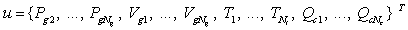(18) A Set of state variables The vector of state variables (dependent variables) x includes the real power output of generator at the slack bus Pg1, voltage at load buses Vli with i = 1, …, Nd, reactive power output of generators Qgi with i = 1,…, Ng, and the apparent power flow in transmission lines Sl with l = 1, …, Nl. The vector x can be expressed as follows: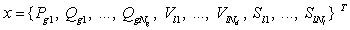(19) Application of Cuckoo Search algorithms for Solving MO-OPF-HTS problem a. Initialization Like the application of CSA to the MO-HTS problem, each nest d in the initial population corresponding to a vector of independent variables is represented as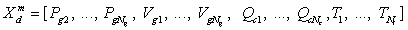where m is the index of optimal subintervals and is from 1 to (M-1) where M is the number of optimal subintervals. Clearly, in the first (M- 1) subintervals the active power of from the second thermal units to the last thermal units and all hydro units are included in each nest in addition to other control variables in the vector u in eq. (18). At the Mth optimal subinterval, the nest d is composed of almost components in Xdm excluding active power of hydro units. The initialization for each nest is as below.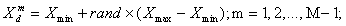(20)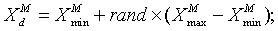(21) where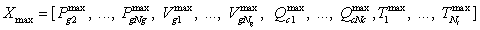(22)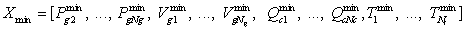(23)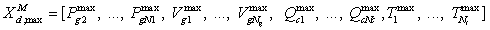(24)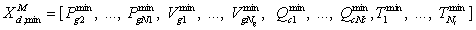(25) The active power from hydro units at generation buses at the Mth subinterval is obtained by using (7) as follows.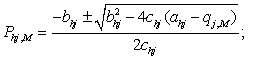(26) where qj,M is the water discharge of the jth hydropower plant at the final optimal subinterval and calculated by: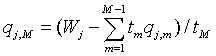(27) The fitness function is regarded as an objective that must be minimized in the CSA and other meta-heuristic algorithms. The fitness function is comprised of objective function such as cost, emission and power loss, and amount of penalty for dependent variables including real power generation at the slack bus, reactive power outputs at the generation buses, load bus voltages, and apparent power flow in transmission lines. The fitness function is determined as the following expression.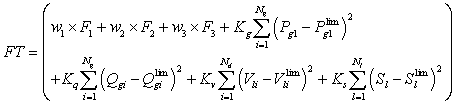(28) where Kg, Kq, Kv, and Ks are penalty factors for active power at slack bus, reactive power generations, load bus voltages, and power flow in transmission lines, respectively; w1, w2 and w3 are respectively weight factors for cost objective, emission objective and total power loss objective. The weight factors are must exactly meet the following equality and inequality constraints: w1+w2+w3=1 (29)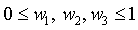(30) where w1, w2 and w3 are weight factors corresponding to fuel, emission and power loss objectives. The limitation of these dependent variables in fitness function is defined as follows.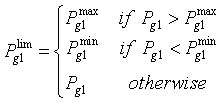(31)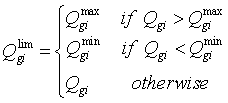(32)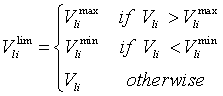(33)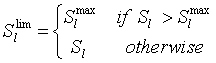(34) Based on the value of fitness function for each nest, the best nest with lowest value of fitness function is set to Gbest while the remaining nests are set to Xbestd b. The first new solution generation via Lévy flights The first new solution generation via Lévy flights is obtained as below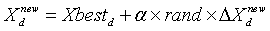(35) where α and the increased value,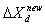are obtained as described in chapter 3. The new solutions will be redefined as eqs. (36) if they violate limitations.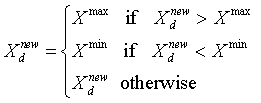(36) Fitness function (28) is then calculated and the entire nest is set to Xbestd c. The second new solution generation via discovery of alien eggs In this section, the second new solution generation is carried out in order to improve the quality of the previously obtained solution. Like the process in MO-HTS problem, in the MO-OPF-HTS problem the action of alien eggs discovery in the nests with a probability of pa is also employed to generate a new solutions and the values of Pa is also within the range from 0 to 1 with a change of 0.1 for each case. The way for obtaining solution is determined by: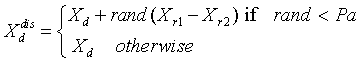(37) The newly obtained solutions also need to be redefined using (36) and the fitness value is calculated using equation (28), and the nest corresponding to the best fitness function is set to the best nest Gbest and the remaining nests are set to Xbestd. d. Termination criteria The computing procedure is terminated as the maximum number of iterations is reached. Numerical Results The applied CSA method is coded in Matlab 7.2 programming language and run on an Intel 1.8 GHz PC with 4 GB of Ram. For each study case, the method is run fifty independent trials. To validate the performance of the applied CSA, anther meta-heuristic algorithm, Particle swarm optimization algorithm, is also implemented for solving the considered problem. a. Selection of control parameters The control parameters of each algorithm are selected as follows. CSA control parameters: The number of nests and the maximum number of iteration are respectively fixed at 12 and 300 for each value of Pa which is ranged from 0 to 1 with a change of 0.1. PSO control parameters: The number of particles and the maximum number of iterations are set to 20 and 300, and two other parameters, c1 and c2 are set to 2.05 b. Obtained results on IEEE 30 bus system The test system comprises 30 buses as in Figure 1 with 6 generation buses, 24 load buses, and 41 branches. The generators are connected at buses 1, 2, 5, 8, 11, and 13 and 4 transformers are located at branches 11, 12, 15, and 36. The system has 2 switchable capacitor bank located at buses 10 and 24. The number of control variables for the system is 17 including real power output of 5 generators except the generator at the slack bus, voltage at 6 generation buses, tap changer of 4 transformers, and reactive power output of 2 switchable capacitor banks. For hydrothermal system, the generators at buses 1, 2, 5, and 8 are driven by steam turbines and the generators, which are working at buses 11 and 13, are run by hydro turbines. The optimal power flow for the hydrothermal system is scheduled in 2 twelve-hour subintervals. The detail of data for the problem is as below. The limitations of active power and reactive power of hydro units and thermal units are shown in Table 1. The coefficients of cost function and emission function of thermal units are respectively given in Tables 2 and 3. Table 4 shows characteristic of hydro generation and available water for two hydropower plants. The limitations of capacitors located at buses 10 and 24 are given in Table 5 and the maximum capacity of transmission line limits is finally given in Table 6. The total data of the IEEE 30-bus system and the coefficients of fuel cost for the thermal units are taken from Power generation, operation and control (John Wiley and Sons) . The coefficients of emission for the thermal units are taken from the coefficients of hydro generation i.e.; Artificial immune system for fixed head hydrothermal power system . The problem of multi-objective optimal power flow for hydrothermal system is divided into two subcases as follows. 1) Minimization of Fuel cost. 2) Minimization of emission. Note that the power losses mentioned in (28) is neglected therefore the weight factor w3 is not included in the two cases. As fuel cost is independently minimized only and emission is neglected, that mean minimization of fuel cost objective F1 is carried out corresponding to the setting of w1 to 1 and w2 to zero. Similarly, as w2 is set to 1 and w1 is set to zero, the individual minimization of emission is performed and the fuel cost is not considered. The best fuel cost corresponding to independent minimization of fuel cost and the best emission corresponding to independent minimization of emission obtained by PSO and CSA are given in Table 7. As seen from the fuel cost, CSA obtains lower cost and lower emission than PSO meanwhile the execution time for searching optimal solution by the two methods is approximate. The fitness function characteristic for economic dispatch case depicted in Figure 2 indicates that the PSO can find out better solution than CSA from the beginning to the iteration of approximately 180 and then the CSA is superior to PSO since its fuel cost is lower than that of PSO. At the end of the search process, the CSA obtains the cost of \$ 15451.2462 while PSO achieves \$ 15457.5459. The Figure is also the same for emission dispatch case shown in Figure 3. In fact, the emission of the PSO is still lower than that of CSA from the start of search procedure until the iterations of proximately 200 and then the emission of PSO is higher than that of CSA and the Figure still last to the end of the search. The optimal solutions obtained by the CSA and PSO are reported in Tables 8 and 9. Conclusion In this paper, the application of Cuckoo Search algorithms to the problem of multi-objective optimal power flow for hydrothermal system is presented. The problem of MO-OPF-HTS is first developed in the study to construct a practical problem in power system that there have not been studies so far. The MO-OPF-HTS is a combination of the optimal power flow problem and the optimal scheduling of hydrothermal system problem where all real constraints in the first problem, which have not been considered in the second problem are taken into account and the hydraulic constraints from the hydropower plants in the second problem are considered. In addition, optimal scheduled subintervals in the second problem are also taken into consideration as well. Consequently, The MO-OPFHTS problem becomes more realistic than the first and the second subproblems. Meta-heuristic algorithms, which are suggested to solve the problem, are PSO and CSA. The obtained results in terms of fuel cost and emission from the methods are respectively for economic dispatch corresponding to minimization of only fuel cost and emission dispatch corresponding to only emission minimization. The comparison has revealed that CSA is very efficient for the problem because it has obtained better fuel cost for economic dispatch and better emission for emission dispatch. The obtained results have indicated that the CSA is a very efficient method for solving the practical problem of MO-OPFHTS. References
Select your language of interest to view the total content in your interested language

### Article Usage

• Total views: 10008
• [From(publication date):
April-2016 - Dec 11, 2019]
• Breakdown by view type
• HTML page views : 9664Can't read the image? click here to refresh